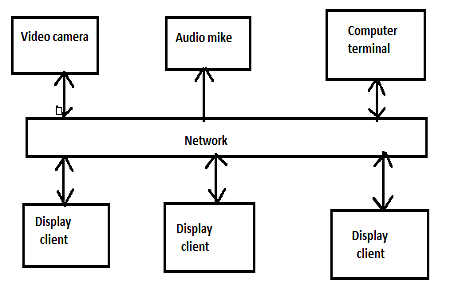• Astrophysics• Electricity• Electromagnetism• Energy• Fields• Force• Mechanics• Momentum• Nuclear Physics• Quantities & Units• States of Matter• Waves• Key ExperimentsTime dilation is a fascinating concept that emerges when we measure elapsed time and simultaneity in space. It's a significant relativistic effect that's worth exploring. To understand it better, we should study why it occurs and what factors we need to consider when measuring space and time accurately. So, what is time dilation? It's a phenomenon that affects how time passes in different parts of space. By taking into account the measurement of elapsed time and simultaneity, we can better understand this effect.

## What are 'time dilation' and 'proper time'?

Time dilation is a mind-bending concept that suggests time is measured differently for moving objects for stationary objects as they travel through space. This phenomenon happens when an observer moves relative to another observer, causing time in their frame of reference to flow more slowly. Proper time refers to the time measured by an observer at rest, relative to the event being observed. An accelerated will measure a shorter elapsed time than a non-accelerated clock between the same two occurrences. We can see this effect in action with spacecraft. For example, for every 12 Earth months, only 0.01 seconds elapse on the International Space Station.

## How can you calculate time dilation?

In order to calculate time dilation, first, you need to imagine which variables resemble which quantities. In order to do that, take a look at the example below.A diagram showing the two views, (a) is the view from the train, and (b) is the view from the platform

Let's consider the paths followed by light as seen by each observer. The observer inside the train sees the light travel straight across and back with a total distance of  2L. The observer on the platform sees the light travel a total distance of 2s  since the train is moving at a velocity v to the right relative to the platform.

We know that the light travels at a speed c = 3.00 ⋅ 108 relative to any observer and the time is distance divided by speed. So the time measured by the observer inside the train is

And it is the proper time. The time observed by the observer on the platform will be

We have found that Δt is equal to  2s / c . In order to place it into the equation, we need to multiply the right side of the equation by  2  and divide it by  c :

Squaring both sides and writing  d  as  vt / 2

Notice how  4L² / c²   equals (Δt 0) ²

If we solve it for Δt

this then gives us

Now if we take the square root on both sides it will yield a simple relation between elapsed times.

Where

## What is an example of time dilation?

Time dilation is a fascinating concept that may seem like it can't be seen in real life since it occurs at relative speeds. However, with today's technology, humans interact with space more than ever before, and we can see the effects of time dilation in action. A great example of this is the global positioning system (GPS). GPS signals travel at relativistic speeds, which means that we need to make corrections for time dilation; otherwise, the GPS system would fail in minutes. The Frisch-Smith experiment is another excellent example of time dilation. This measured a quantity of muons emitted by the sun per unit time. The results showed that approximately 73% of muons reached the endpoint per hour because their proper time is smaller than the proper time of an observer on Earth. This confirmed that time was flowing more slowly for them with a time dilation factor of 8.8.

Time dilation is the concept that time is measured differently for moving objects than for stationary objects as they travel through space. Proper time is the time measured by an observer at rest, relative to the event being observed. Time dilation occurs when one observer moves relative to another observer, causing time to flow more slowly. As we interact more with space through technology like GPS, calculations for time dilation are becoming increasingly necessary in daily life.

## Time Dilation

What is time dilation?

Time dilation is the concept that time is measured differently for moving objects than for stationary objects as they travel through space.

How do you calculate time dilation?

In order to find the relation between proper time and the time observed you need to use the equations of time dilation.

Does time dilation have an effect on our daily lives?

Yes it does. Since technology has evolved in such a way that we are connected with space more than ever before. One of the best examples for this is GPS, since it relies on signals traveling at relativistic speeds and time dilation corrections need to be done.14-day free trial. Cancel anytime.Join 10,000+ learners worldwide.The first 14 days are on us96% of learners report x2 faster learningFree hands-on onboarding & supportCancel Anytime